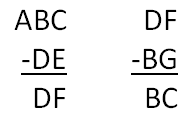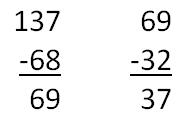# Extra Hard SAT Math Question – Way Too Many Variables

At the end of every SAT Math section, the test makers try to come up with an extremely difficult problem that will leave even the cleverest students scratching their heads. The really evil part, though, is that even these problems can be solved in under a minute without a calculator – if you know what to do. This means that once you “figure out the trick,” these difficult problems become easy. So, while those test makers are busy cackling with sadistic glee, let’s see if we can’t beat them at their own game.

Consider the following problem:In the correctly solved subtraction problems above, each of the seven letters represents a different single digit integer. What is the average (arithmetic mean) of DE and BG?

A) 32

B) 50

C) 68

D) 100

E) 137

“How on Earth am I supposed to figure out the average of DE and BG if I have no idea what any of those seven numbers are?” Well, you could try the guess and check method, but that would waste a lot of time. If you think about it, you will realize that you don’t actually need to know what all seven integers are in order to solve this problem: you only need to figure out one.

What we have in the first subtraction problem is a three digit number that is the sum of two two digit numbers. A two digit number must be less than 100 by definition. 100 + 100 = 200, so the two two digit numbers added together must be less than 200, since each of them alone is less than 100. Thus, the three digit number must be between 99 and 200. Therefore, the letter A must be the number 1.

If A represents 1, then we can rewrite ABC as:

ABC = 100 + BC

The one other thing you need to realize is that we can get rid of DF. Write out each problem horizontally:

ABC – DE = DF

DF – BG = BC

In the second one, isolate DF:

DF = BC + BG

Now, it becomes clear that you can set the two equations equal to each other:

ABC – DE = DF = BC + BG

ABC – DE = BC + BG

Now substitute 100 + BC for ABC:

(100 + BC) – DE = BC +BG

100 + BC = BC + BG + DE

100 = BG + DE

The BC appears on both sides of the equation, so it cancels out, and you are left with the realization that the sum of BG and DE is 100. Finding their average is now elementary (as Sherlock Holmes might say):